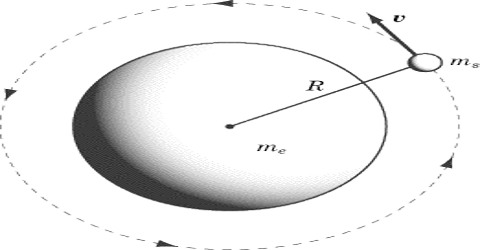# Gravitation

Gravitation

Gravity, also called gravitation, is a force that exists among all material objects in the universe. Famous scientist Sir Isaac Newton discovered that between any two particles in the universe there exists a force of attraction. This attractive force between the two particles is sometimes called gravitation and sometimes gravity. There is a difference between these two forces. Then question arises: what is gravitation and gravity?

Gravitation: The force of attraction between two objects or particles in the universe is called gravitation.

Gravity: The force of attraction between the earth and any other object or particle is called gravity.

Examples: The attractive force between the sun and the moon is called gravitation, whereas the force of attraction between the earth and the moon is called gravity.